Solution of Gravitation (Page No - 103 & 104) - Physics by Lakhmir Singh, Class 9

# Solution of Gravitation (Page No - 103 & 104) - Physics by Lakhmir Singh, Class 9 - Extra Documents & Tests for Class 9

Question 71:
If the distance between two masses is increased by a factor of 5, by what factor would the mass of one of them have to be altered to maintain the same gravitational force ? Would this be an increase or decrease in the mass ?
Solution :
Gravitational force is given by: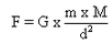Distance between two masses is increased  s.t new distance is D = 5d

New Gravitational force F1 = F

Let on of the mass is changed to mso as to maintain the same gravitational force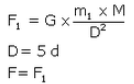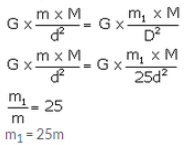Hence the mass should be increased by 25 times in order to have the same gravitational force.

Question 72:
Universal law of gravitation states that every object exerts a gravitational force of attraction on every other object. If this is true, why don’t we notice such forces ? Why don’t the two objects in a room move towards each other due to this force ?The universal law of gravitation states that every object exerts a gravitational force of attraction on every other object. if this is true, then why don't we see the various objects in a room moving toward one another?

Solution :
In order to be able to notice the gravitational force of attraction between any two objects, at least one of the objects on the earth should have an extremely large mass. Since no object on the earth have an extremely large mass, we cannot notice such forces.
The two objects in a room do not move towards each other because due to their small masses, the gravitational force of attraction between them is very, very weak.

Question 73:
Suppose a planet exists whose mass and radius both are half those of the earth. Calculate the acceleration due to gravity on the surface of this planet.
Solution :
Acceleration due to gravity of earth,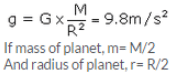Acceleration due to gravity  on the surface of planet will be :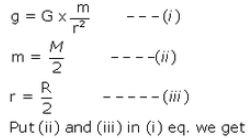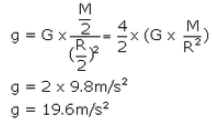Lakhmir Singh Physics Class 9 Solutions Page No:104

Question 74:
A coin and a piece of paper are dropped simultaneously from the same height. Which of the two will touch the ground first ? What will happen if the coin and the piece of paper are dropped in vacuum ? Give reasons for your answer.
Solution :
The coin reaches the ground first as compared to the piece of paper because it experiences lesser resistance from air than that felt by paper.
If the coin and the piece of paper are dropped in vacuum, both of them will touch the ground at the same time.

Question 75:
A stone and the earth attract each other with an equal and opposite force. Why then we see only the stone falling towards the earth but not the earth rising towards the stone ?
Solution :
The mass of a stone is very small, due to which the gravitational force produces a large acceleration in it. Due to large acceleration of stone, we see stone falling towards the earth. The mass of earth is, however, very, very large. Due to the very large mass of the earth, the same gravitational force produces very, very small acceleration in the earth, that it cannot be observed. And hence we do not see the earth rising up towards the stone.

Question 76:
What is the actual shape of the orbit of a planet around the sun ? What assumption was made by Newton regarding the shape of an orbit of a planet around the sun for deriving his inverse square rule from Kepler’s third law of planetary motion ?
Solution :
The actual shape of the orbit of a planet around the sun is elliptical. The assumption made by the Newton regarding the shape of an orbit of a planet around the sun was that the orbit of a planet around the sun is ‘circular’.

Question 77:
The values of g at six distances A, B, C, D, E and F from the surface of the earth are found to be 3.08 m/s2, 9.2.3 m/s2, 0.57 m/s2, 7.34 m/s2, 0.30 m/s2 and 1.49 m/s2, respectively.
(a) nArrange these values of g according to the increasing distances from the surface of the earth (keeping the value of g nearest to the surface of the earth first)
(b) If the value of distance F be 10000 km from the surface of the earth, state whether this distance is deep . inside the earth or high up in the sky. Give reason for your answer.

Solution :
(a) 9.23 m/s2 , 7.34 m/s2 , 3.08 m/s2 , 1.49 m/s2 , 0.57 m/s2 , 0.30 m/s2
(b) This distance F of 10000 km is high up in the sky. The distance of 10000 km cannot be deep inside the earth because the radius of earth is only about 6400km and the value of g at the centre of earth becomes zero.

The document Solution of Gravitation (Page No - 103 & 104) - Physics by Lakhmir Singh, Class 9 | Extra Documents & Tests for Class 9 is a part of the Class 9 Course Extra Documents & Tests for Class 9.
All you need of Class 9 at this link: Class 9

## Extra Documents & Tests for Class 9

1 videos|228 docs|21 tests

## FAQs on Solution of Gravitation (Page No - 103 & 104) - Physics by Lakhmir Singh, Class 9 - Extra Documents & Tests for Class 9

 1. What is the equation for gravitational force between two objects?Ans. The equation for gravitational force between two objects is given by Newton's law of universal gravitation, which states that the gravitational force (F) between two objects is directly proportional to the product of their masses (m1 and m2) and inversely proportional to the square of the distance (r) between their centers of mass. Mathematically, it can be represented as F = G * (m1 * m2) / r^2, where G is the gravitational constant.
 2. How does the gravitational force depend on the masses of the objects?Ans. The gravitational force between two objects is directly proportional to the product of their masses. This means that as the mass of one or both objects increases, the gravitational force between them also increases. Similarly, if the mass of one or both objects decreases, the gravitational force between them decreases.
 3. What is the significance of the gravitational constant (G) in the equation for gravitational force?Ans. The gravitational constant (G) is a fundamental constant in physics that determines the strength of the gravitational force. It is a universal constant and its value is approximately 6.67430 × 10^(-11) N*m^2/kg^2. The value of G is crucial in calculating the gravitational force between two objects. Without this constant, it would not be possible to accurately determine the strength of the force.
 4. How does the distance between two objects affect the gravitational force between them?Ans. The gravitational force between two objects is inversely proportional to the square of the distance between their centers of mass. This means that as the distance between the objects increases, the gravitational force between them decreases. Conversely, if the distance between the objects decreases, the gravitational force between them increases. The relationship is such that doubling the distance between two objects will result in the gravitational force being reduced to one-fourth of its original value.
 5. How does the gravitational force affect the motion of objects in the universe?Ans. The gravitational force plays a crucial role in the motion of objects in the universe. It is responsible for holding planets in their orbits around the Sun, moons around planets, and satellites around the Earth. The force of gravity also governs the motion of celestial bodies, such as stars, galaxies, and even clusters of galaxies. Without the gravitational force, these objects would not be held together and their motion would be completely different. The gravitational force provides the necessary centripetal force for objects to maintain their circular or elliptical orbits.

## Extra Documents & Tests for Class 9

1 videos|228 docs|21 testsExplore Courses for Class 9 examSignup to see your scores go up within 7 days! Learn & Practice with 1000+ FREE Notes, Videos & Tests.
10M+ students study on EduRev
Track your progress, build streaks, highlight & save important lessons and more!
Related Searches

,

,

,

,

,

,

,

,

,

,

,

,

,

,

,

,

,

,

,

,

,

,

,

,

;